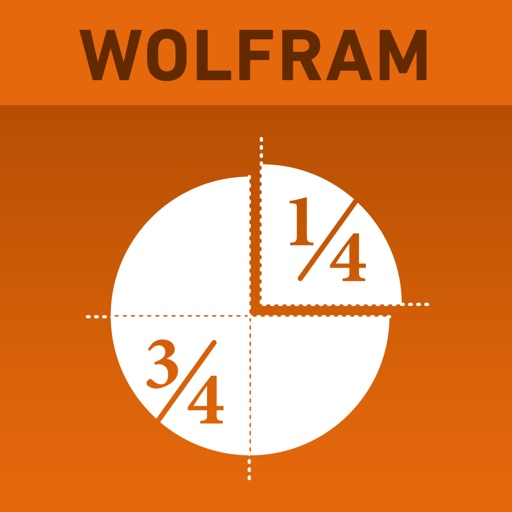## Learning fractions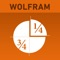# Wolfram Fractions Reference App

by Wolfram Group LLC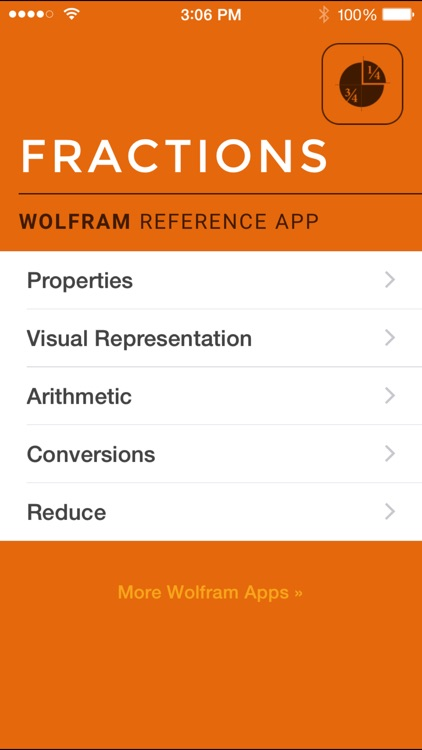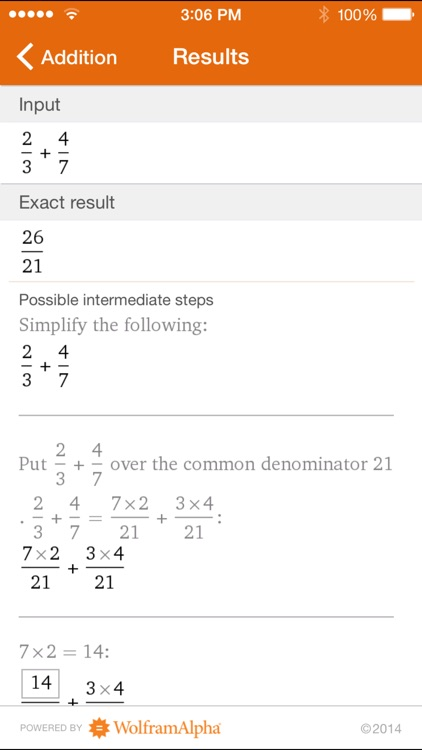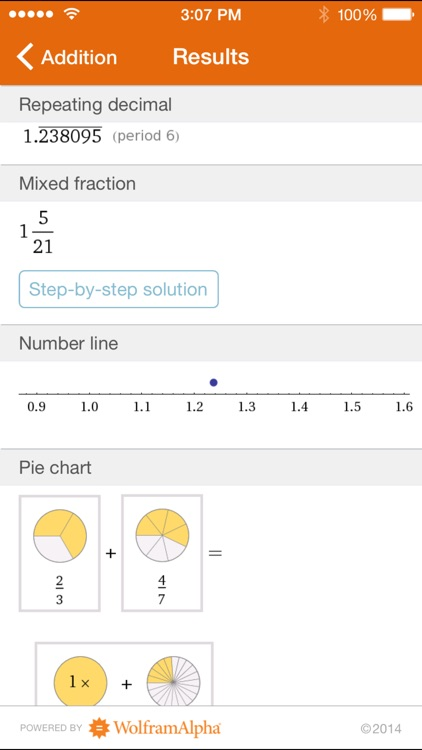Learning fractions? Then you need the Wolfram Fractions Reference App. Whether adding, subtracting, or converting fractions, the Wolfram Fractions Reference App will help you with your specific fraction problems.### App Details

Version
1.3.1
Rating
(1)
Size
11Mb
Genre
Reference Education
Last updated
May 15, 2017
Release date
June 30, 2012

### App Screenshots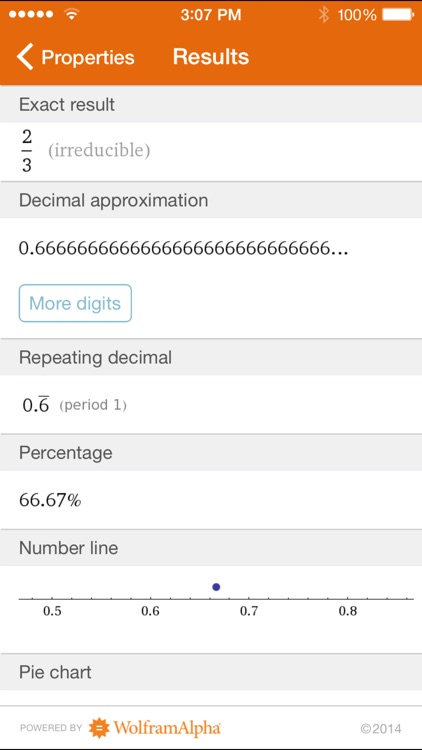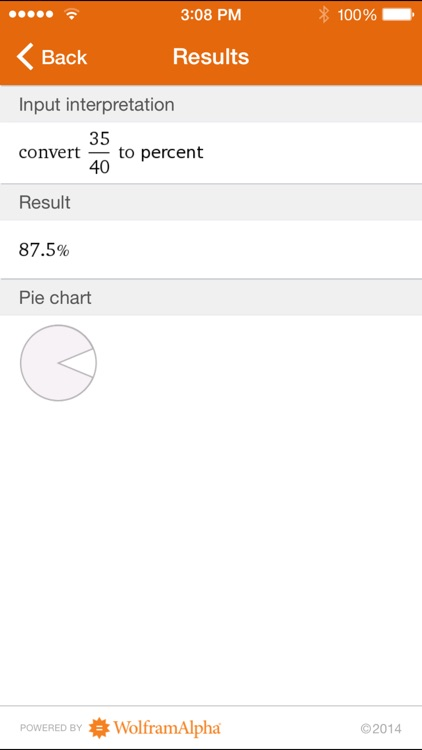### App Store Description

Learning fractions? Then you need the Wolfram Fractions Reference App. Whether adding, subtracting, or converting fractions, the Wolfram Fractions Reference App will help you with your specific fraction problems.

- Visualize fractions on a number line or pie chart
- Convert a fraction to a decimal or percent, or vice versa
- Get help with arithmetic including addition, subtraction, multiplication, and division
- Reduce fractions to their simplest forms